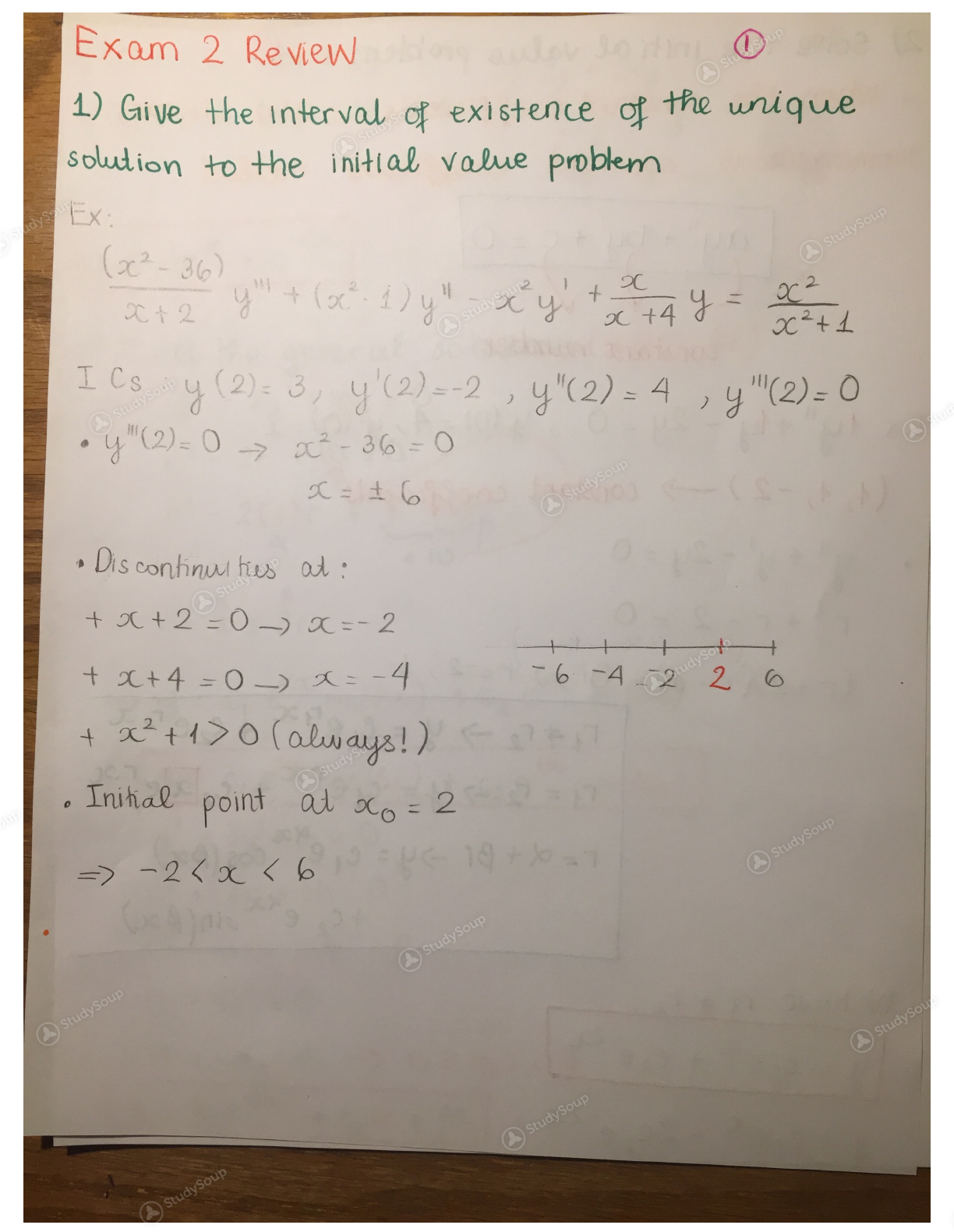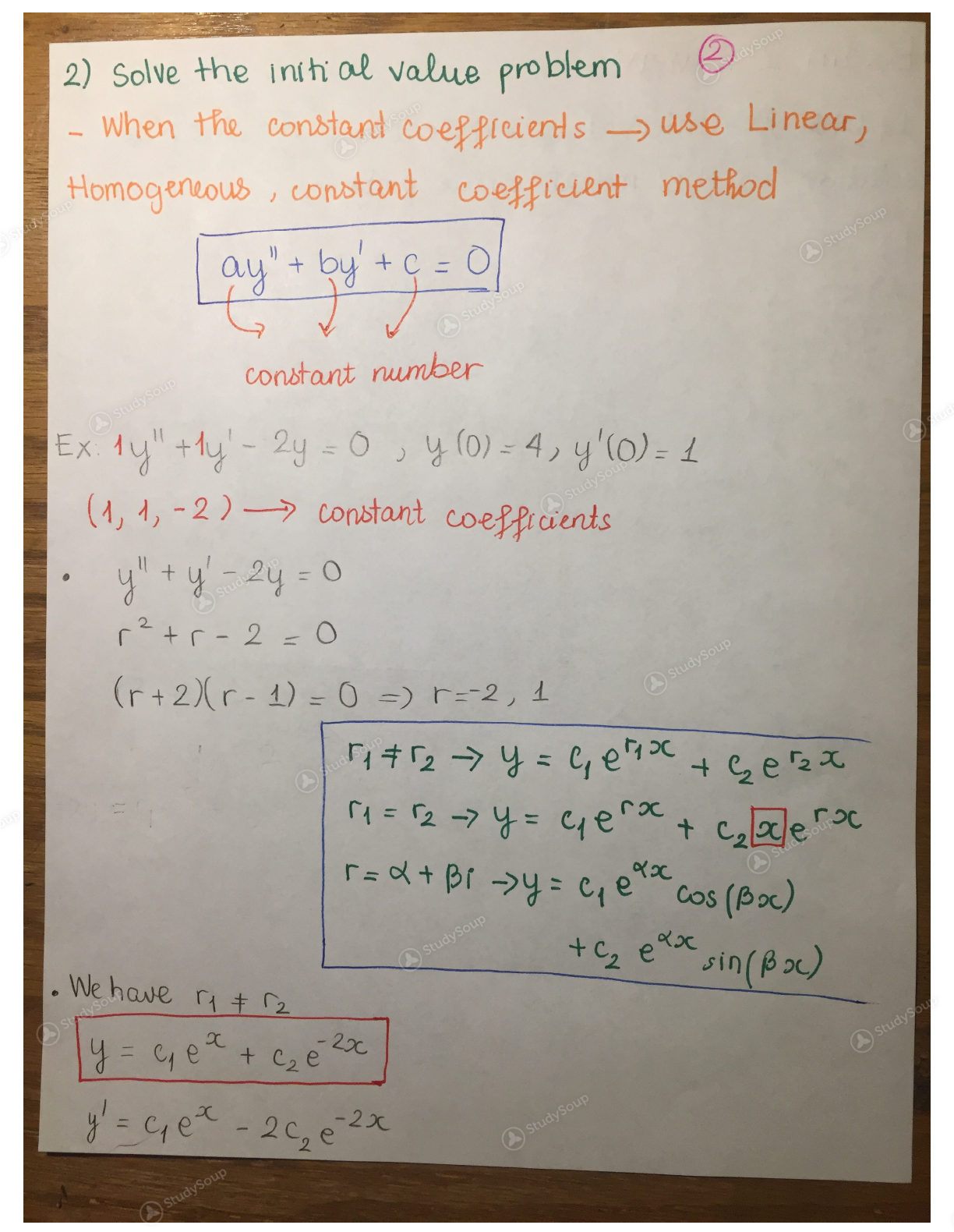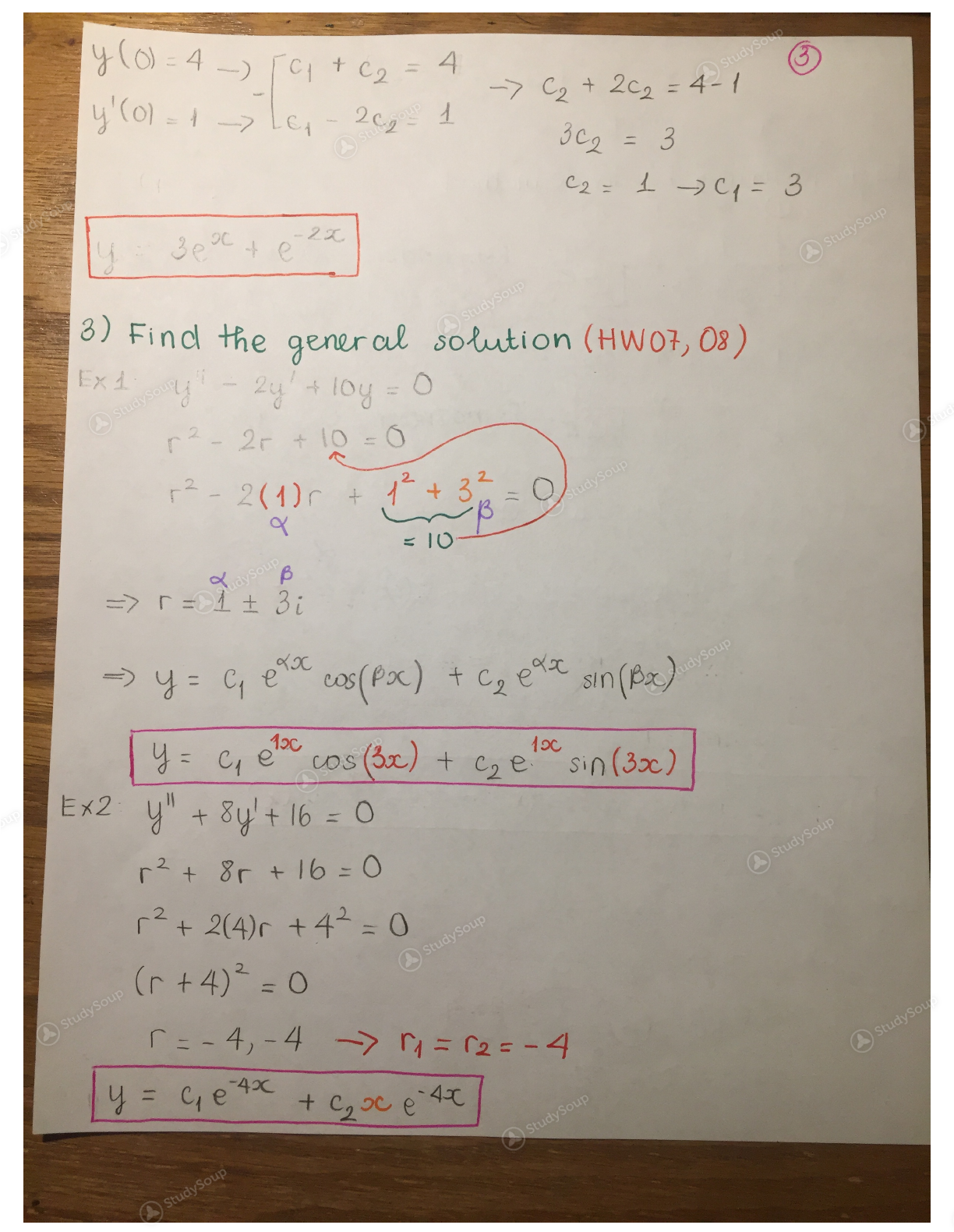Limited time offer 20% OFF StudySoup Subscription details

TTU - MATH 3350 - MATH 3350_ EXAM 2 Review - Study Guide

Created by: Tram Nguyen Elite Notetaker

> > > > TTU - MATH 3350 - MATH 3350_ EXAM 2 Review - Study Guide

TTU - MATH 3350 - MATH 3350_ EXAM 2 Review - Study Guide

Description: This is the review for exam 2 on this Friday. This exam will be difficult if you don't prepare well. In each method that we learned in the class, I highlight the important information and also did some examples. If something is not clear, please send me an email, I would like to help.
This preview shows pages 1 - 3 of a 3 page document. to view the rest of the contentThis is the end of the preview. Please to view the rest of the contentJoin more than 18,000+ college students at Texas Tech University who use StudySoup to get ahead
3 Pages 32 Views 25 Unlocks
• Better Grades Guarantee
• 24/7 Homework help
• Notes, Study Guides, Flashcards + More!Join more than 18,000+ college students at Texas Tech University who use StudySoup to get ahead
Description: This is the review for exam 2 on this Friday. This exam will be difficult if you don't prepare well. In each method that we learned in the class, I highlight the important information and also did some examples. If something is not clear, please send me an email, I would like to help.
3 Pages 32 Views 25 Unlocks
• Better Grades Guarantee
• 24/7 Homework help
• Notes, Study Guides, Flashcards + More!

Unformatted text preview: Exam 2 Review 1) Give the interval of existence of the unique solution to the initial value problem A (x-36 12 y - xy +244 9 = ICs y (2): 3, y'(2) --2 , y"(2) = 4 , y "(2) = 0 y"(2): 0 - x - 36 - 0 -6 -4.2 2 Dis conhnui ftes od: + 3 + 2 = 0 3 =- 2 + 2+4 = 0 _) a = -4 + x +1>0 (always!) . Initial point ad a. - 2 => -24x < 6 19 Al ShadySoup A Study soul Study o Study soup Study Soup2) Solve the Inih al value pro blem e - When the constant coefficients > use Linear, Homogeneous , constant coefficient method A saysoup Lay' + by' + c = D constant number Ex. ly" +ly' - 2y = 0 , 4 (0) - 4, y'(o) = 1 |(1,1, - 2 ) constant coefficients y" + y' - 2y = 0 r +r - 2 = 0 (r+2)(r - 1) = 0 =) r=-2, 1 4 + 2 = 0, 09 >' + y ex = P2 -> y = ye"x + c,aera r= * + Br ->y = ct exacos (Pac) +c, ex sin(B 5) . We have C2 y = 9e + cp E 20 y' = get - 2c, e-2x Study A Study Soup study soup4 (0) - 4 - C + C2 = A y'(0) = 1 -> le, 209 - 1 -> C + 202 = 4-1 32 = 3 C2 : 1 - = 3 30, e 22 3) Find the gener al solution (HW07, 08 ) r2 - 2(1) + L= 0 =>r = 1 + 3i (A study sour -> y = C ea cos(PX) + C, et sin (br) y = C, e cos (2x) + ca eto s:0 (3x) E 2 y" + 8y'+ 16 = 0 r + 8r + 16 = 0 r + 24) +42 = 0 (r +4)2 = 0 C = -4,-4 n = 2-4 y = C4 4x + ca 20 e 40 A study soup A studyson udy soup Study Soup

Get Full Access to TTU - MATH 3350 - Study Guide - Midterm
×
Get Full Access to TTU - MATH 3350 - Study Guide - Midterm

I don't want to reset my password

Need help? Contact support

Need an Account? Is not associated with an account
We're here to help

Having trouble accessing your account? Let us help you, contact support at +1(510) 944-1054 or support@studysoup.com

Password Reset Request Sent An email has been sent to the email address associated to your account. Follow the link in the email to reset your password. If you're having trouble finding our email please check your spam folder
Already have an Account? Is already in use